## Using Linear, Square, and Cubic Measure Appropriately

### Learning Outcomes

• Given an item to measure determine whether you would use linear, square, or cubic units
• Calculate perimeter and area of a rectangle

When you measure your height or the length of a garden hose, you use a ruler or tape measure (see below). A tape measure might remind you of a line—you use it for linear measure, which measures length. Inch, foot, yard, mile, centimeter and meter are units of linear measure.

This tape measure measures inches along the top and centimeters along the bottom.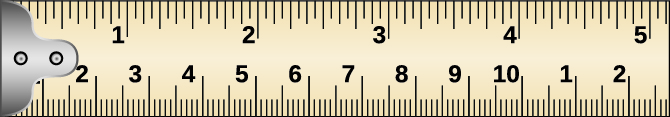When you want to know how much tile is needed to cover a floor, or the size of a wall to be painted, you need to know the area, a measure of the region needed to cover a surface. Area is measured is square units. We often use square inches, square feet, square centimeters, or square miles to measure area. A square centimeter is a square that is one centimeter (cm) on each side. A square inch is a square that is one inch on each side (see below).

Square measures have sides that are each $1$ unit in length.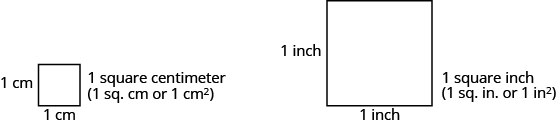The next image shows a rectangular rug that is $2$ feet long by $3$ feet wide. Each square is $1$ foot wide by $1$ foot long, or $1$ square foot. The rug is made of $6$ squares. The area of the rug is $6$ square feet.

The rug contains six squares of $1$ square foot each, so the total area of the rug is $6$ square feet.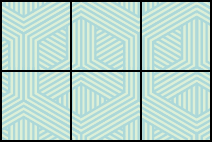When you measure how much it takes to fill a container, such as the amount of gasoline that can fit in a tank, or the amount of medicine in a syringe, you are measuring volume. Volume is measured in cubic units such as cubic inches or cubic centimeters. When measuring the volume of a rectangular solid, you measure how many cubes fill the container. We often use cubic centimeters, cubic inches, and cubic feet. A cubic centimeter is a cube that measures one centimeter on each side, while a cubic inch is a cube that measures one inch on each side (see below).

Cubic measures have sides that are $1$ unit in length.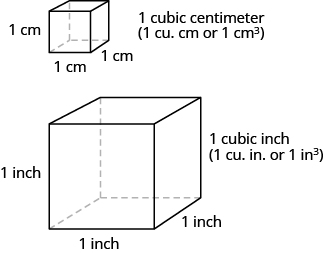Suppose the cube in the image below measures $3$ inches on each side and is cut on the lines shown. How many little cubes does it contain? If we were to take the big cube apart, we would find $27$ little cubes, with each one measuring one inch on all sides. So each little cube has a volume of $1$ cubic inch, and the volume of the big cube is $27$ cubic inches.

A cube that measures $3$ inches on each side is made up of $27$ one-inch cubes, or $27$ cubic inches.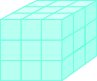### example

For each item, state whether you would use linear, square, or cubic measure:
1. amount of carpeting needed in a room
2. extension cord length
3. amount of sand in a sandbox
4. length of a curtain rod
5. amount of flour in a canister
6. size of the roof of a doghouse.

Solution

 1. You are measuring how much surface the carpet covers, which is the area. square measure 2. You are measuring how long the extension cord is, which is the length. linear measure 3. You are measuring the volume of the sand. cubic measure 4. You are measuring the length of the curtain rod. linear measure 5. You are measuring the volume of the flour. cubic measure 6. You are measuring the area of the roof. square measure

### try it

Many geometry applications will involve finding the perimeter or the area of a figure. There are also many applications of perimeter and area in everyday life, so it is important to make sure you understand what they each mean.

Picture a room that needs new floor tiles. The tiles come in squares that are a foot on each side—one square foot. How many of those squares are needed to cover the floor? This is the area of the floor.

Next, think about putting new baseboard around the room, once the tiles have been laid. To figure out how many strips are needed, you must know the distance around the room. You would use a tape measure to measure the number of feet around the room. This distance is the perimeter.

### Perimeter and Area

The perimeter is a measure of the distance around a figure.
The area is a measure of the surface covered by a figure.

The image below shows a square tile that is $1$ inch on each side. If an ant walked around the edge of the tile, it would walk $4$ inches. This distance is the perimeter of the tile.

Since the tile is a square that is $1$ inch on each side, its area is one square inch. The area of a shape is measured by determining how many square units cover the shape.

$\begin{array}{c}\text{Perimeter}=4\text{ inches}\\ \text{Area}=1\text{ square inch}\end{array}$

When the ant walks completely around the tile on its edge, it is tracing the perimeter of the tile. The area of the tile is $1$ square inch.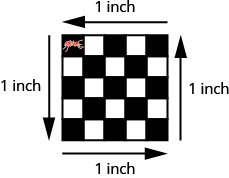### example

Each side of two square tiles is $1$ square inch. Two tiles are pushed side by side and shown together below.
1. What is the perimeter of the figure?
2. What is the area?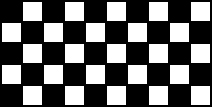### try it

In the following video we show a similar example as the ones above.